• 主要介绍了利用keras使用神经网络预测销量操作，具有很好的参考价值，希望对大家有所帮助。一起跟随小编过来看看吧
• from keras.datasets import boston_housing from keras import models from keras import layers (X_train, y_train), (X_test, y_test) = boston_...#对数据进行标准化预处理，方便神经网络更好的学习 mean = X_...
from keras.datasets import boston_housing
from keras import models
from keras import layers

(X_train, y_train), (X_test, y_test) = boston_housing.load_data()#加载数据

#对数据进行标准化预处理，方便神经网络更好的学习
mean = X_train.mean(axis=0)
X_train -= mean
std = X_train.std(axis=0)
X_train /= std
X_test -= mean
X_test /= std

#构建神经网络模型
def build_model():
#这里使用Sequential模型
model = models.Sequential()
#进行层的搭建，注意第二层往后没有输入形状(input_shape)，它可以自动推导出输入的形状等于上一层输出的形状
#编译网络
model.compile(optimizer='rmsprop', loss='mse', metrics=['mae'])
return model

num_epochs = 100
model = build_model()
model.fit(X_train, y_train,epochs=num_epochs, batch_size=1, verbose=0)
predicts = model.predict(X_test)

在实际操作中可以用自己的数据进行测试，最终预测出的predicts，可以利用回归评价指标和y_test进行模型效果的评价。
展开全文python
• Ubuntu中利用h5py保存训练好的keras神经网络模型；h5py詳細安装；
Ubuntu中利用h5py保存训练好的keras神经网络模型
利用h5py保存的模型所占的空间非常小。在利用h5py保存keras训练好的模型之前需要先安装h5py，具体安装过程詳細如下。

（1）利用h5py保存和读取keras模型的代码如下：
import h5py from keras.models import model_from_json
json_string = model.to_json()
open('my_model_architecture.json','w').write(json_string)
model.save_weights('my_model_weights.h5')
#读取model
model.load_weights('my_model_weights.h5')（2）安装h5py步驟：
直接利用 sudo pip install h5py 首先出现没有cython；安装完cython后会提示一个g++错误，这是由于没有安装hdf5；安装完hdf5再安装h5py就能够成功安装。
安装h5py的命令如下：
sudo pip install cython
sudo apt-get install libhdf5-dev
sudo pip install h5py
安装完成后可以用如下命令測試：
userT@t5810:~\$ python
Python 2.7.6 (default, Oct 26 2016, 20:30:19)
[GCC 4.8.4] on linux2
>>>
>>> import h5py
>>>

轉載自：
http://blog.csdn.net/linmingan/article/details/50736300
http://blog.csdn.net/linmingan/article/details/50736615


展开全文• 我是一个python小白，最近在学习一些有关神经网络的知识： 偶然之间发现了一本有关keras的书还不错，有详细的代码，我也不知道该怎么学习keras，先从分析代码开始，内容会有点啰唆，大神轻喷。以下代码引自《keras...
我是一个python小白，最近在学习一些有关神经网络的知识：
偶然之间发现了一本有关keras的书还不错，有详细的代码，我也不知道该怎么学习keras，先从分析代码开始，内容会有点啰唆，大神轻喷。以下代码引自《keras深度学习实战》
from __future__ import print_function
import numpy as np
from keras.datasets import mnist
from keras.models import Sequential
from keras.layers.core import Dense, Activation
from keras.optimizers import SGD
from keras.utils import np_utils

np.random.seed(1671)  # 重复性设置

# 网络和训练
NB_EPOCH = 200
BATCH_SIZE = 128
VERBOSE = 1
NB_CLASSES = 10   # 输出个数等于数字个数
OPTIMIZER = SGD() # SGD 优化器
N_HIDDEN = 128
VALIDATION_SPLIT=0.2 # 训练集中用作验证集的数据比例

#数据：混合并划分训练集和测试集
#
(X_train, y_train), (X_test, y_test) = mnist.load_data()

#X_train 是 60000 行 28x28 的数据 --> 变形为 60000 x 784
RESHAPED = 784
#
X_train = X_train.reshape(60000, RESHAPED)
X_test = X_test.reshape(10000, RESHAPED)
X_train = X_train.astype('float32')
X_test = X_test.astype('float32')

# 归一化处理
#
X_train /= 255
X_test /= 255
print(X_train.shape, 'train samples')
print(X_test.shape, 'test samples')

# 将类向量转换为二值类别矩阵
Y_train = np_utils.to_categorical(y_train, NB_CLASSES)
Y_test = np_utils.to_categorical(y_test, NB_CLASSES)

# 10个输出
# 最后是softmax激活函数

model = Sequential()

model.summary()  #打印出模型概况，它实际调用的是keras.utils.print_summary

model.compile(loss='categorical_crossentropy',
optimizer=OPTIMIZER,
metrics=['accuracy'])

history = model.fit(X_train, Y_train,
batch_size=BATCH_SIZE, epochs=NB_EPOCH,
verbose=VERBOSE, validation_split=VALIDATION_SPLIT) #verbose 是日志显示
score = model.evaluate(X_test, Y_test, verbose=VERBOSE)
print("\nTest score:", score)
print('Test accuracy:', score)

上面的代码用Keras定义一个识别MNIST手写数字的网络，利用一个简单的神经网络最后训练出来的效果其实还挺好的。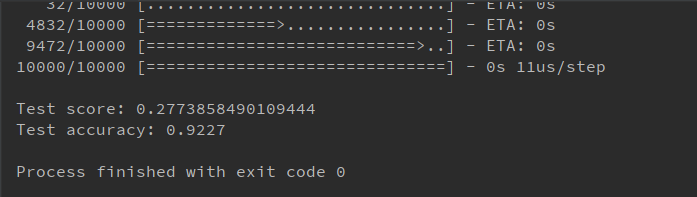利用keras构建神经网络模型其实很简洁
1.模块导入
#
from __future__ import print_function
import numpy as np
from keras.datasets import mnist
from keras.models import Sequential
from keras.layers.core import Dense, Activation
from keras.optimizers import SGD
from keras.utils import np_utils

咱们一行一行解释这些代码是干啥用的？
from __future__ import print_function

加上这句话后，即使在python2.X，使用print就得像python3.x那样加括号使用。python2.X中print不需要括号，而在python3.X中则需要。如果是python3.X版本，这句话加不加都没影响。
import numpy as np

导入numpy库，NumPy(Numerical Python) 是 Python 语言的一个扩展程序库，支持大量的维度数组与矩阵运算，此外也针对数组运算提供大量的数学函数库。
from keras.datasets import mnist
from keras.models import Sequential
from keras.layers.core import Dense, Activation
from keras.optimizers import SGD
from keras.utils import np_utils

第一行是从keras.datasets加载手写数字数据集,keras主要有7种，详细介绍可以参考这位大神写的博客 https://blog.csdn.net/weixin_41770169/article/details/80249986
第三行 layer是层，Dense是全连接层，Activation是激活层
第四行是优化器optimizer, 这个模型使用的是SGD随机梯度下降优化算法
第五行 这个的功能是实现编码输出标签。


展开全文• 利用keras搭积木，快速搭建出一个神经网络模型，训练鸢尾花分类 代码 import numpy as np import pandas as pd import matplotlib.pyplot as plt from scipy.io import loadmat from sklearn.model_selection import...
利用keras搭积木，快速搭建出一个神经网络模型，训练鸢尾花分类
代码
import numpy as np
import pandas as pd
import matplotlib.pyplot as plt
from sklearn.model_selection import train_test_split
import keras
from keras.models import Sequential
from keras.layers import Dense

# 导入数据
path = '../Data/iris.data'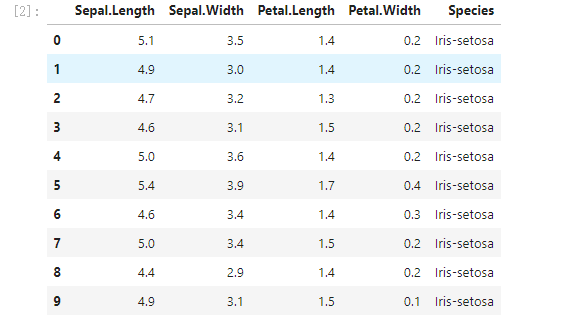# 映射函数iris_type: 将string的label映射至数字label
def iris_type(s):
class_label = {'Iris-setosa':0, 'Iris-versicolor':1, 'Iris-virginica':2}
return class_label[s]
Data = pd.read_csv(path,names=['Sepal.Length','Sepal.Width','Petal.Length','Petal.Width','Species'], converters = {4:iris_type})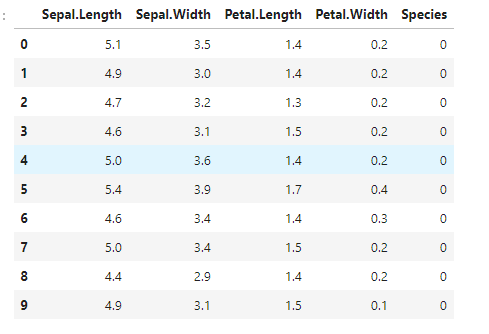# 变量初始化
# 最后一列为y，其余为x
cols = Data.shape #列数 shape行数 列数
X = Data.iloc[:,0:cols-1]       #取前cols-1列，即输入向量
y = Data.iloc[:,cols-1:cols]    #取最后一列，即目标变量
X = np.array(X)
y = np.array(y)
y = y.flatten()       # 对y进行降维

def realdata(y,k):
real = np.zeros(shape=(k,len(y)))
for i in range(0,k):
y_i = np.array([1 if label == i else 0 for label in y])
real[i] = y_i
return real.T
y = realdata(y,3)
y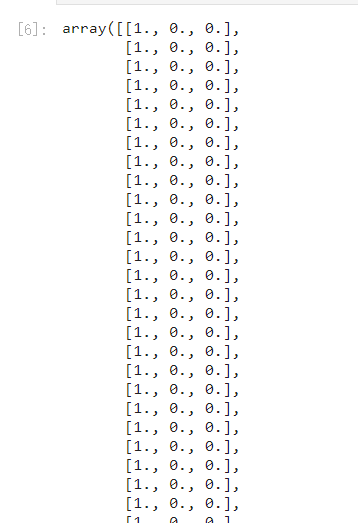X_train,X_test,y_train,y_test = train_test_split(X,y,test_size=0.3)
#y_train=y_train.T
X_train.shape,y_train.shape

# 搭建神经网络
# 搭建神经网络
model = Sequential()
model.add(Dense(units=5, activation='relu',input_dim = 4))   # 输入层，5个激活单元，激活函数为relu，输入数据维度为（4，）

model.compile(loss='categorical_crossentropy', optimizer='sgd',metrics=['accuracy'])

model.fit(X_train,y_train,batch_size=1,epochs = 20)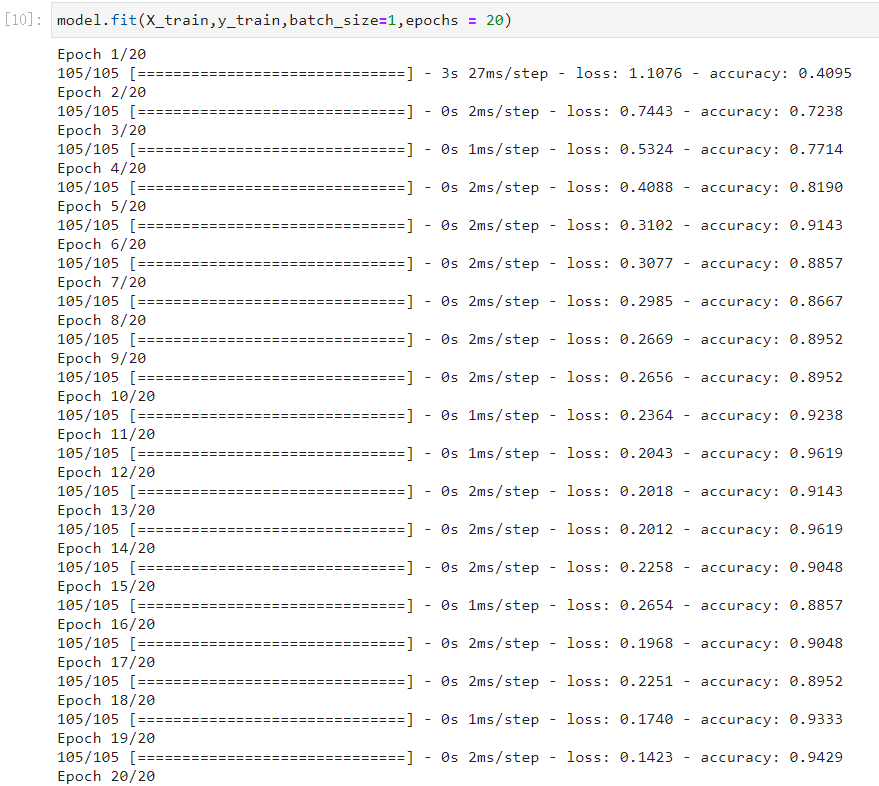result = model.predict(X_test)
np.round(result,2)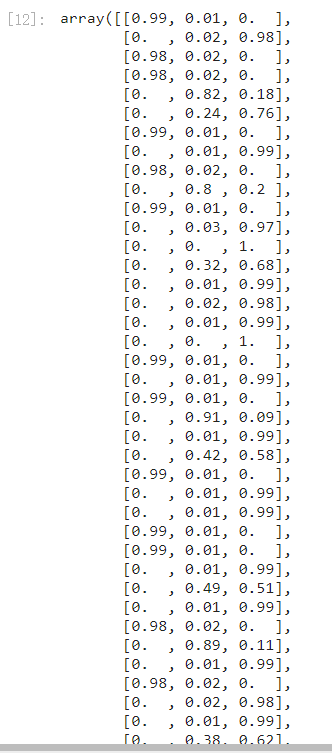score = model.evaluate(X_test,y_test)
print('loss值为：',score)
print('准确率为：',score)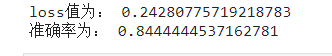展开全文深度学习 python tensorflow 机器学习
• 神经网络学习小记录7——利用Keras进行CNN网络构建学习前言Keras中构建CNN的重要函数1、Conv2D2、MaxPooling2D3、metrics=['accuracy']全部代码 学习前言 利用Keras构建完普通BP神经网络后，还要会构建CNN。 Keras...深度学习
• Recurrent Shop：利用Keras构建复杂的递归神经网络的框架
• 利用基于tensorflow2的keras框架，搭建CNN卷积神经网络模型，对手写数字识别数据集mnist进行分类，网络规模小，训练精度高。网络包括三个卷积层，两个池化层和全连接层，在测试集上实现了99%左右的识别率。
• 神经网络就是一个简单的lstm神经网络，调用了keras模块，现在想比较不同训练方法的效率，如何在训练完成后显示每一步训练以及最后训练完的总时间？深度学习
• Keras中提供了一个神经网络可视化的函数plot，并可以将可视化结果保存在本地 from keras.utils.vis_utils import plot_model plot_model(model, to_file='model.png') 安装： 首先安装pydot模块（直接利用conda ...Windows 可视化tensorflow 人工智能 机器学习 深度学习
• 一旦你利用Keras完成了训练，你可以将你的网络保存在HDF5里面。 今天毛利灭了它 只需要导入from keras.models import model_from_json就是这么简单 基于Iris数据集 from sklearn import datasets import numpy as np...
• 有一种观点：利用股票历史股价数据，搭建神经网络深度学习，预测股票未来走势，是外行人士的发神经。原因不外乎这些：首先，现有的量价指标等分析工具，远比仅研究历史股价数据靠谱；其次，涨停板的限制、新股N个...python 深度学习 爬虫 数据分析
• 神经网络学习小记录8——利用Keras进行简单RNN网络构建学习前言Keras中构建RNN的重要函数1、SimpleRNN2、model.train_on_batch全部代码 学习前言 还要会RNN噢。 Keras中构建RNN的重要函数 1、SimpleRNN SimpleRNN...RNN
• 利用h5py保存的模型所占的空间非常小。在利用h5py保存keras训练好的模型之前需要先安装h5py，具体的安装过程可参考我关于...利用h5py保存和读取keras模型的代码如下：   import h5py from keras.models import m...h5py python ubuntu
• 前言该项目通过卷积神经网络加Q-learning算法，利用Keras框架共210行代码实现了让程序自己学习如何玩耍FlappyBird。安装依赖库pip install keras pip install pygame pip install scikit-image pip install h5py如果...深度学习 框架 算法
• AI：Keras神经网络全连接层多分类问题的训练损失、验证损失和训练精度、验证精度，Python import keras from keras.layers import Dense from keras import models from keras import optimizers from sklearn....AI 人工智能 机器学习 PythonPython
• 直接上代码 from PIL import Image ...from keras.utils import to_categorical path="F:\\kaggle\\dog_vs_cat\\" train_X=np.empty((2000,227,227,3),dtype="float16") train_Y=np.empty((2000,),dtyp...
• 神经网络学习小记录6——利用Keras进行简单分类学习前言Keras中分类的重要函数1、np_utils.to_categorical2、Activation3、metrics=['accuracy']全部代码 学习前言 上一步讲了如何构建回归算法，这一次将怎么进行...分类 手写体识别
• 正则项在优化过程中层的参数或层的激活值添加惩罚项，这些惩罚项将与损失函数一起作为网络的最终优化目标 惩罚项基于层进行惩罚，目前惩罚项的接口与层有关，但Dense, Conv1D, Conv2D, Conv3D具有共同的接口。...
• win10下基于anaconda利用keras开展16系显卡GTX1650的GPU神经网络计算 虽然安装了双系统，但ubantu的确是不太常用，所以还是尝试一下win10下的GPU神经网络计算。从实践看，大体步骤与ubantu下一致，下面进行详细介绍...python
• Keras是一个神经网络开发的高级API，用Python编写，底层调用神经网络开发库TensorFlow、CNTK或Theano。 Keras的目标是简化神经网络应用开发过程，快速实现想法。 Keras 后端 Keras 是一个模型级库，为开发深度学习...python 深度学习
• 欢迎进入TensorFlow2.0入门到进阶的第二章内容，这一章将主要讲解利用tf.keras的实战部分。本节主要是对tf.keras的简要介绍。 文章目录1、理论部分1.1 TensorFlow-Keras简介1.1.1 Keras1.1.2 tf-keraskeras相同点...python 机器学习 人工智能 深度学习
• 神经网络学习小记录9——利用Keras进行模型的保存与读取学习前言Keras中构建RNN的重要函数1、model.save;2、load_model. ## 1、model.save model.save用于保存模型，在保存模型前，首先要利用pip install安装h5py的...hdf5
• # -*- coding: utf-8 -*- """ ...#利用卷积神经网络进行手写数字识别 from keras.models import Sequential from keras.layers import Dense,Dropout,Flatten from keras.la...python 深度学习
• 如何利用Keras中的权重约束减少深度神经网络中的过拟合 权重约束提供了一种方法，用于减少深度学习神经网络模型对训练数据的过度拟合，并改善模型对新数据（例如测试集）的性能。有多种类型的权重约束，例如最大和...
• Keras搭建分类神经网络2.1.导入必要模块2.2.数据预处理2.3.搭建模型2.4.激活模型2.5.训练+测试 1.前言 今天用 Keras 来构建一个分类神经网络，用到的数据集是 MNIST，就是 0 到 9 这几个数字的图片数据集。 2.用...分类 数据挖掘...